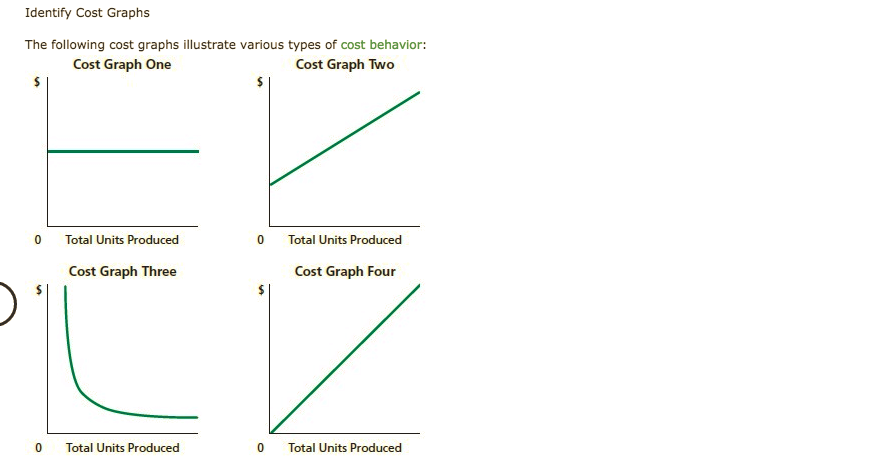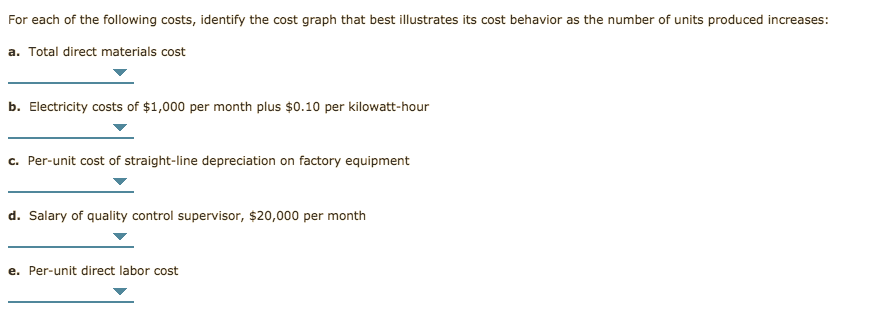# Identify Cost Graphs The following cost graphs illustrate various types of cost behavior: Cost Graph One Cost Graph Two 0 Total Units Produced 0 Total Units Produced Cost Graph Three Cost Graph Four 0 Total Units Produced Total Units Produced For each of the following costs, identify the cost graph that best illustrates its cost behavior as the number of units produced increases: a. Total direct materials cost b. Electricity costs of $1,000 per month plus$0.10 per kilowatt-hour c. Per-unit cost of straight-line depreciation on factory equipment d. Salary of quality control supervisor, $20,000 per month e. Per-unit direct labor cost Questionhelp_outlineImage TranscriptioncloseIdentify Cost Graphs The following cost graphs illustrate various types of cost behavior: Cost Graph One Cost Graph Two 0 Total Units Produced 0 Total Units Produced Cost Graph Three Cost Graph Four 0 Total Units Produced Total Units Produced fullscreenhelp_outlineImage TranscriptioncloseFor each of the following costs, identify the cost graph that best illustrates its cost behavior as the number of units produced increases: a. Total direct materials cost b. Electricity costs of$1,000 per month plus $0.10 per kilowatt-hour c. Per-unit cost of straight-line depreciation on factory equipment d. Salary of quality control supervisor,$20,000 per month e. Per-unit direct labor cost fullscreen

### Want to see the step-by-step answer?